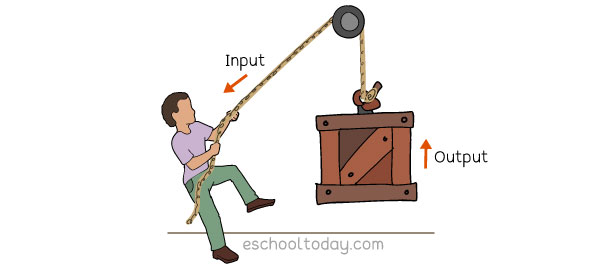## Important Simple Machine terms

Lever
A solid bar that rotates around a pivot and makes it easier to lift a load, move a heavy object, or apply a force.

Force
A force is a push or a pull. The unit of Force is called the Newton. Force (F) = Mass x Acceleration.

Work
The movement of an object resulting from a force applied to it. Movement is usually in the direction of the force. To calculate Work done, multiply Force (F) by Distance (D). W=FxD

Input Force
The effort or force you put into a machine.

Output Force
The force the machine produces as a result of the input force.Power
Power is the rate at which Work is done. Power is measured in Watt. Power = Work / Time